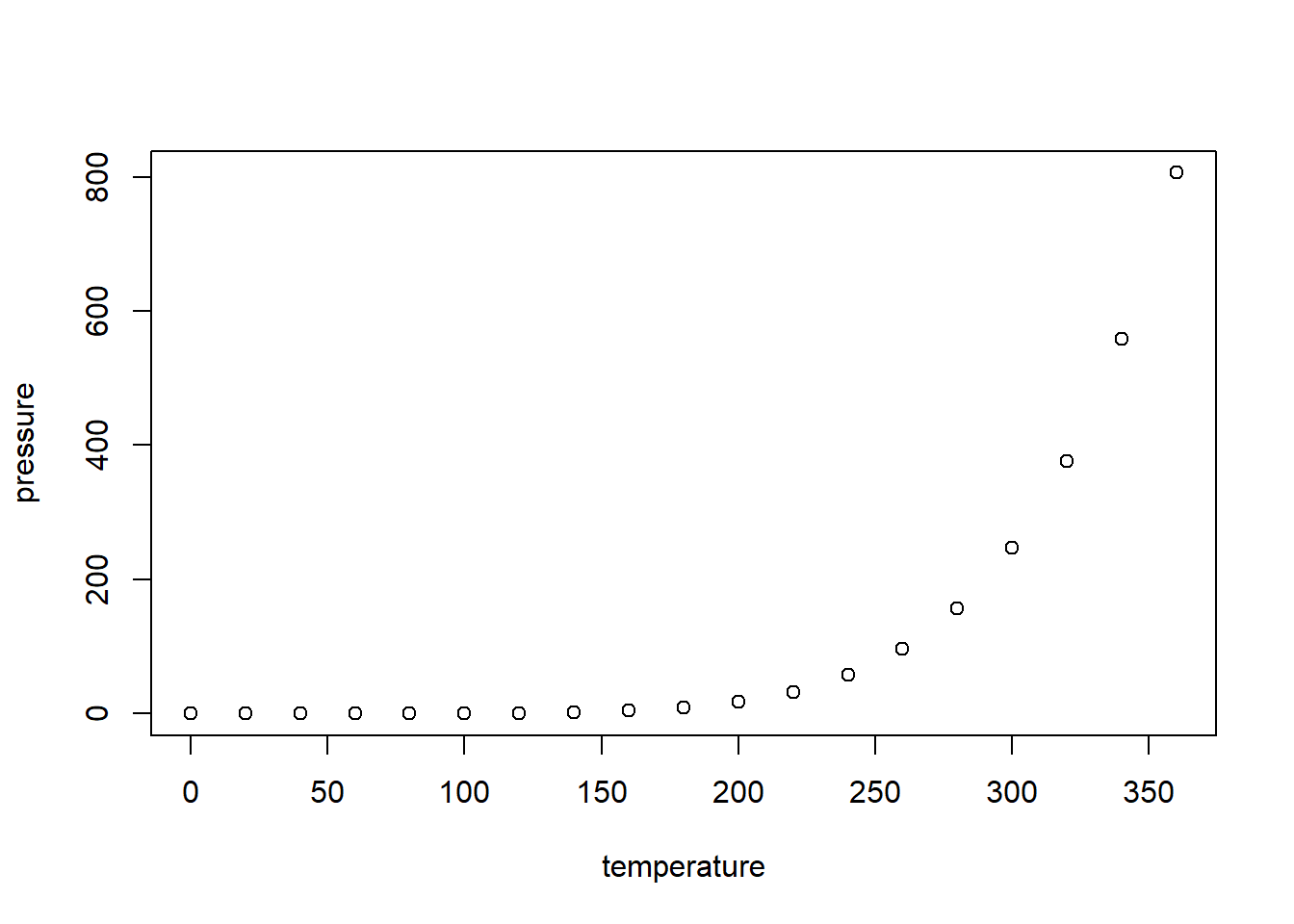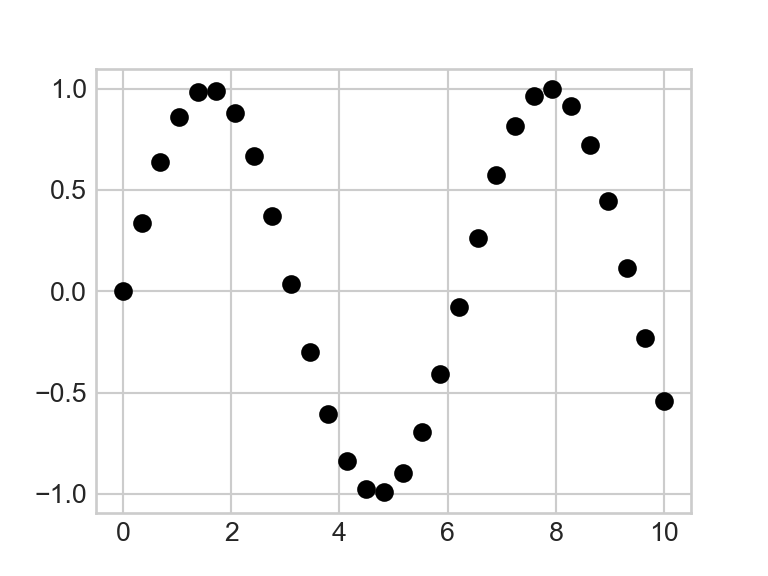## R Markdown Basics

This is an R Markdown document. R Markdown is a simple formatting syntax for authoring HTML, PDF, and MS Word documents (among others!). I recommend using it to create HTML documents, and to instead use LaTeX or knitr (LaTeX with embedded R code) documents to make PDFs (For more on LateX and/or knitr see https://pauljhurtado.com/latex/).

For further information on using R Markdown see http://rmarkdown.rstudio.com, especially the section Get Started. There, you can find various resources including an R Markdown Cheatsheet – I like the older version at https://rstudio.com/wp-content/uploads/2015/02/rmarkdown-cheatsheet.pdf – and the R Markdown Reference Guide https://rstudio.com/wp-content/uploads/2015/03/rmarkdown-reference.pdf.

### Getting Started

In R Studio (https://www.rstudio.com/), you can start a new R Markdown document by going to File > New File > R Markdown. When you click the Knit button a document will be generated that includes both content as well as the output of any embedded R code chunks within the document. We’ll get to that shortly, but first, a little more on basic formatting and equations. To get R and R Studio installed (along side the free software LaTeX) see http://www.pauljhurtado.com/teaching/software.html.

### Text Formatting

View the source file for this document to view examples. To make text bold place a pair asterisks (or underscore characters) before and after the text. To make text italic, put a single asterisk (or underscore character) before and after the text. To format inline (R) code, such as names of funcions and packages, place grave accents (, not apostrophes like ’) on either end of the text. Use multiple # at the start of a line to define a section (## Section Title) and subsection headers (### Subsection Title). If compiling to produce an HTML document, then HTML and CSS can also be included to format text like this or like this.

### Equations

In short, LaTeX works!

$$\frac{dx_1}{dt} = f(x_1)$$


$\frac{dx_1}{dt} = f(x_1)$

Similarly,

\begin{equation}
\frac{dy}{dt}=g(y)
\label{eq:dydt}
\end{equation}


$\begin{equation} \frac{dy}{dt}=g(y) \label{eq:dydt} \end{equation}$

Note that to get the equation numbering for eq. \eqref{eq:dydt} above to work, I had to add the following to the top of the R markdown source file (https://stackoverflow.com/questions/35026405/auto-number-equations-in-r-markdown-documents-in-rstudio):

<script type="text/x-mathjax-config">
MathJax.Hub.Config({
TeX: {
equationNumbers: {
autoNumber: "AMS",
formatNumber: function (n) {return '1.'+n}
}
}
});
</script>

The amsmath package is mostly functional (it’s all run through mathjax by pandoc), for example

$A = \begin{bmatrix} a & b \\ c & d \end{bmatrix}$

Some environments are limited however. For example, wrapping the following in a subequations environment fails.

\begin{align} \frac{dN}{dt} =& \; r\,N\bigg(1-\frac{N}{K}\bigg) - a\,N\,P \\ \frac{dP}{dt} =& \; \chi\,a\,N\,P - \mu\,P \end{align}

## R code and output

You can embed an R code chunk like this:

{r cars}
summary(cars)


This yields the output

summary(cars)
##      speed           dist
##  Min.   : 4.0   Min.   :  2.00
##  1st Qu.:12.0   1st Qu.: 26.00
##  Median :15.0   Median : 36.00
##  Mean   :15.4   Mean   : 42.98
##  3rd Qu.:19.0   3rd Qu.: 56.00
##  Max.   :25.0   Max.   :120.00

### Including Plots

You can also embed plots, for example:

{r pressure, echo=FALSE}
plot(pressure)


yields…### Code Chunk Options

Note that, above, the optional echo = FALSE parameter was added to the code chunk to prevent printing of the R code that generated the plot.

For more on Code Chunk options, see https://rmarkdown.rstudio.com/lesson-3.html and the R Markdown Reference Guide at https://rstudio.com/wp-content/uploads/2015/03/rmarkdown-reference.pdf.

### Other Languages

You can also call other languages, e.g., if you have python installed (Anaconda users need to add the additional lines 4-5 per https://stackoverflow.com/questions/50352614/r-markdown-how-can-i-make-rstudio-display-python-plots-inline-instead-of-in-new/50711837#50711837):

import matplotlib.pyplot as plt
plt.style.use('seaborn-whitegrid')
import numpy as np
import os
os.environ['QT_QPA_PLATFORM_PLUGIN_PATH'] = '/Users/MrStandardUser/Anaconda3/Library/plugins/platforms'

x = np.linspace(0, 10, 30)
y = np.sin(x)

plt.plot(x, y, 'o', color='black')
plt.show()For more on using python in conjunction with R, see https://rstudio.github.io/reticulate/articles/r_markdown.html.

For more on the different “engines” available beyond R and Python, run the following in the R console: names(knitr::knit_engines\$get())`.